Updated date:

# Simple Machines — How Does a Lever Work?

Eugene is a qualified control/instrumentation engineer Bsc (Eng) and has worked as a developer of electronics & software for SCADA systems.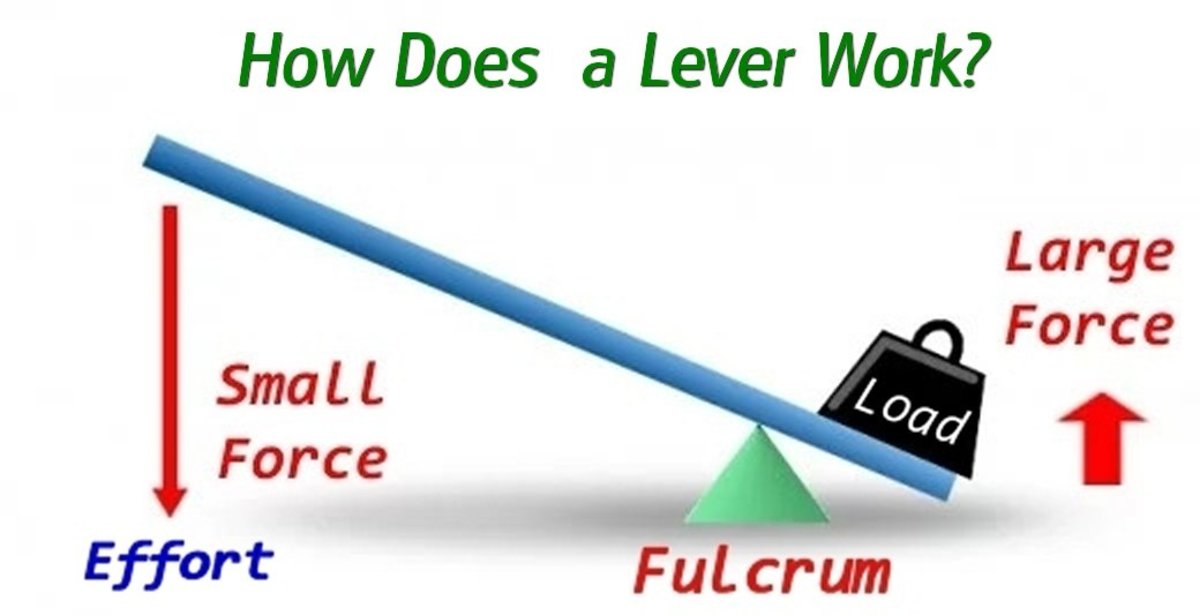A lever can magnify force.Original image public domain, Dr Christopher S. Baird

## The Lever — One of the Six Classical Simple Machines

The lever is one of the six simple machines which were defined by Renaissance scientists hundreds of years ago. The other machines are the wheel, the inclined plane, the screw, the wedge and the pulley. You have used a lever in some shape or form without actually realizing it. So for instance scissors, nut crackers, pliers, hedge shears, bolt cutters and lopping shears all use levers in their design. A prybar or crowbar is a lever also, and when you prise open the lid of a tin with the handle of a spoon, you are using "the law of the lever" to create a greater force. A long handle on a wrench provides more "leverage". A claw hammer also acts as a lever when pulling out nails. A see-saw and wheelbarrow are also levers.

## What is a Force?

To understand how a lever works, we first need to learn about forces. A force can be thought of as a "push" or "pull". A force is required for example to lift a weight or slide it on a surface.

### Examples of forces:

• A forklift lifting a load.
• Tension in a spring when you pull on it.
• A magnet pulling a piece of iron.
• Air in a balloon, football or tire, pushing outwards on it's walls.
• The force of gravity keeping things on the ground.
• Air or water resisting the movement of a car, aircraft or ship. This is called drag.

An active force results in a reactive force, so for instance when you pull on a spring, this is the active force. The tension in the spring is the reactive force pulling back.

## What Does Mechanical Advantage Mean?

A simple machine can magnify a force. The degree to which the force is magnified is called the mechanical advantage. Levers are great because they increase mechanical advantage and can generate much larger forces. For example a hammer or crowbar can easily produce a ton of force for pulling out nails, lifting a rock or prising up boards.

## What are The Parts of a Lever?

• Beam. The physical lever itself made of materials such as wood, metal or plastic which can pivot or move on the fulcrum
• Effort. The force that is exerted by a person or machine on a lever
• Fulcrum. The point at which a lever pivots or hinges
• Load. The object that is acted on by the lever.

## You've Used a Lever Without Knowing It!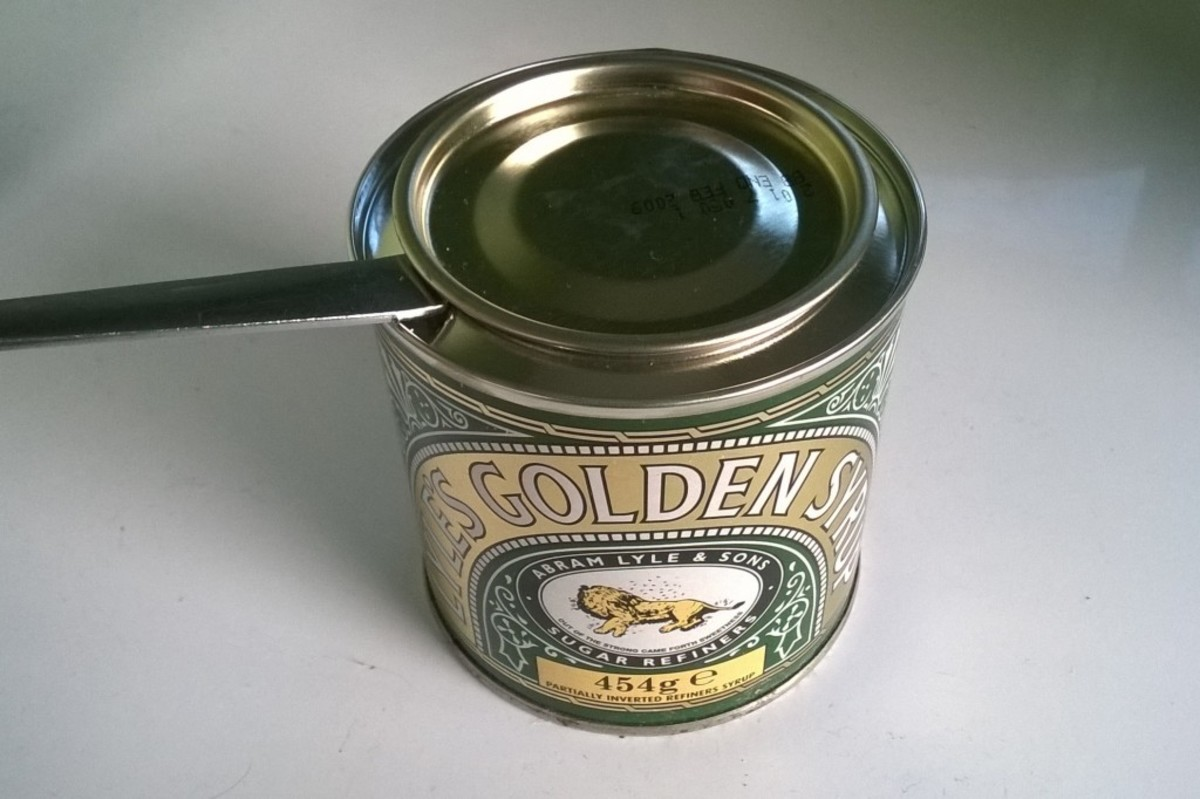Using the handle of a spoon to open a tin. The spoon acts as a lever, creating a larger force to lift the lid. The fulcrum is the rim of the tin© Eugene Brennan

## What are Levers Examples in Everyday Life?

• Crowbars and prybars
• Pliers
• Scissors
• Bottle openers
• Bolt cutters
• Nut crackers
• Claw hammer
• Wheel barrow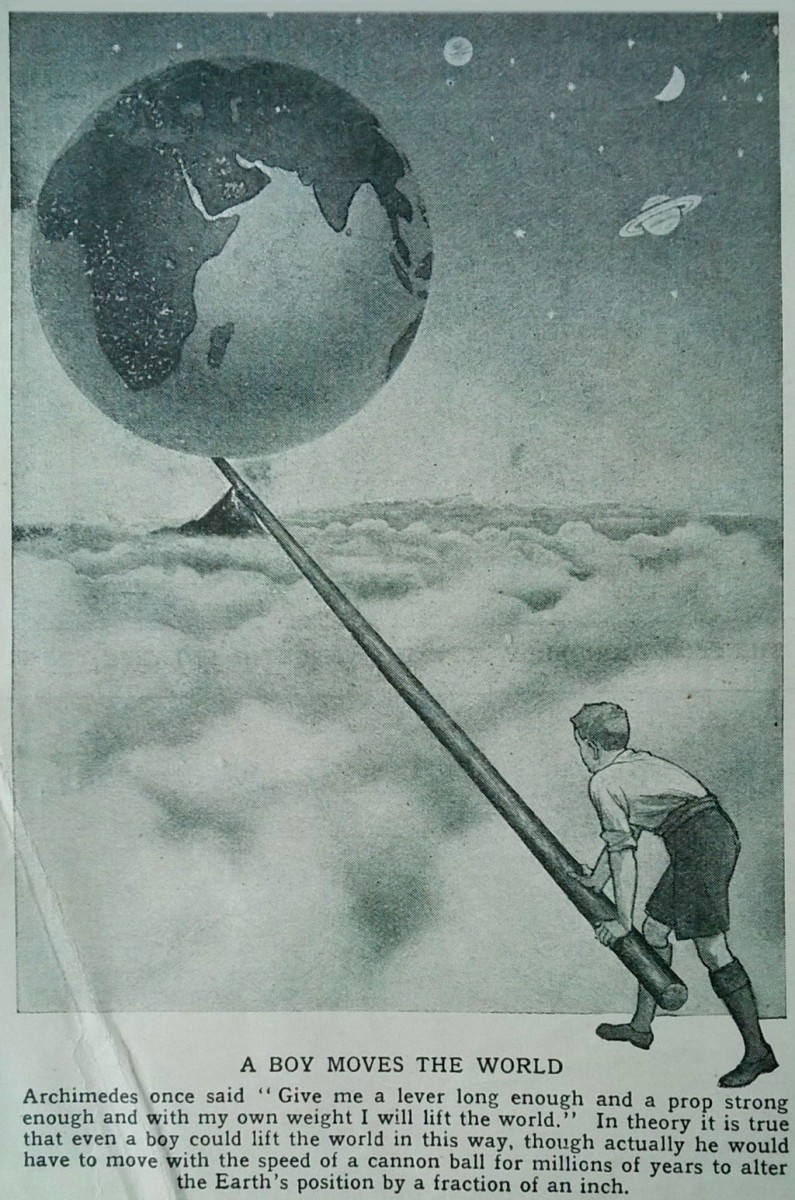From "The World of Wonder" a children's science periodical from the 1930s"The World of Wonder" published circa 1935

## What are the Three Classes of Levers?

The class of a lever depends on the position of the effort, fulcrum and load.

## First Class Lever

The effort is on one side of the lever and the load is on the other side. The fulcrum is in the middle. Moving the fulcrum closer to the load increases the mechanical advantage and increases the force on the load.

### Examples of first class levers:

Scissors, pliers, hammer.

## Second Class Lever

The effort is on one side of the lever and the fulcrum is on the other side with the load between the effort and fulcrum. Keeping the effort in the same position and moving the load closer to the fulcrum, increases the force on the load.

### Examples of second class levers:

Nutcracker and wheelbarrow.

## Third Class Lever

The fulcrum is on one end of the lever, the load is on the other side and the effort is between the load and fulcrum. A third class lever has less of a mechanical advantage than the other two types because the distance from the load to the fulcrum is greater than the distance from the effort to the fulcrum.

### Examples of third class levers:

A human arm, broom, sporting equipment e.g. baseball bat.

## Examples of Levers

Typical examples of levers.Using a crowbar as a lever to lift a heavy piece of stone.Public domain image via Pixabay.com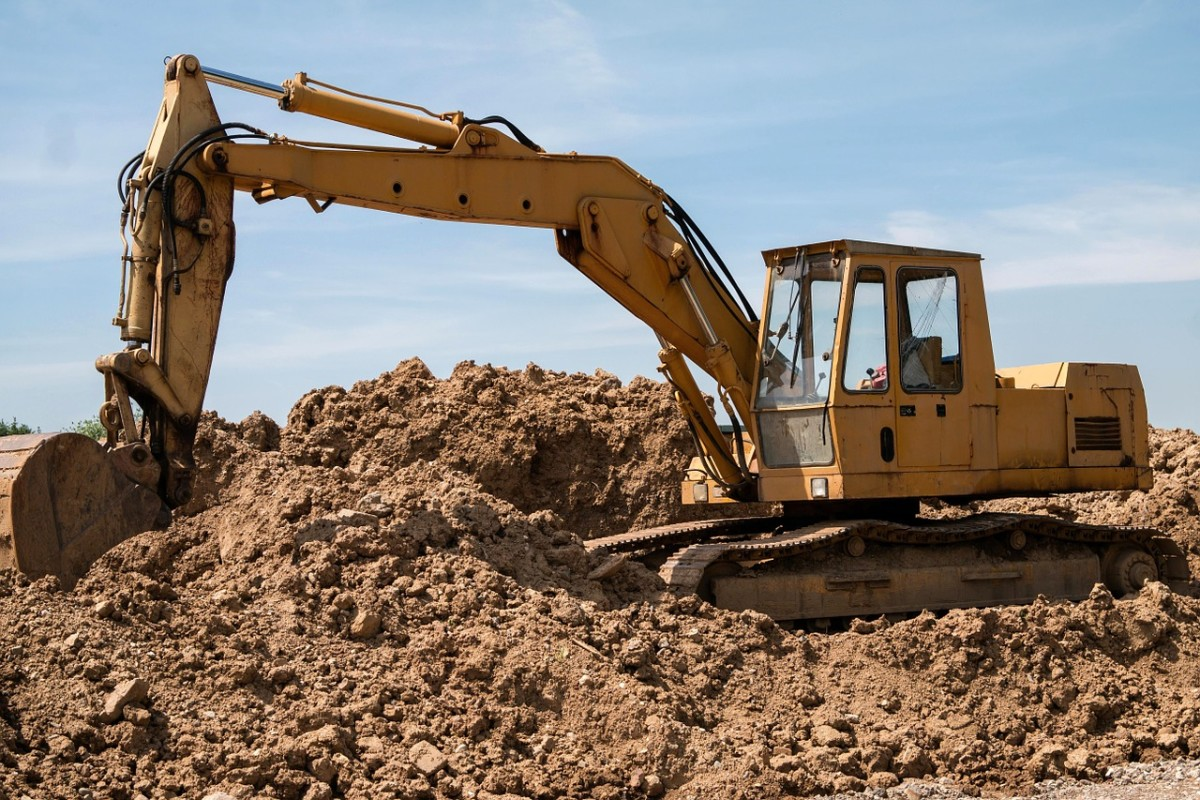An excavator (digger) has several connected levers on its boom. Hydraulic cylinders produce the force required to move the levers.Didgeman, public domain image via Pixabay.com

## What is the Moment of a Force?

To understand how levers work, we first need to understand the concept of moment of a force. The moment of a force about a point is the magnitude of the force multiplied by the perpendicular distance from the point, to the line of direction of the force.

## How Levers Work - The Physics

In the diagram below, two forces act on the lever. This is a schematic or diagram, but it symbolically represents any of the real life levers mentioned above.

The lever pivots at a point called a fulcrum represented by the black triangle (in real life, this could be the screw holding the two blades of a scissors together). A lever is said to be balanced when the lever doesn't rotate and everything is in equilibrium (e.g. two people of equal weight sitting on a see-saw, at equal distances from the pivot point).

In the diagram above, a force F1 acts downward on the lever at a distance d1 from the fulcrum.

When balanced:

"The sum of the clockwise moments equals the sum of the counter-clockwise moments"

Another force F2 at distance d2 from the fulcrum acts downwards on the lever. This balances the effects of F1 and the lever is stationary, i.e. there is no net turning force.

So for F1, the clockwise moment is F1d1

and for F2, the counter-clockwise moment is F2d2

And when the lever is balanced, i.e. not rotating and static, the clockwise moment equals the counter-clockwise moment, so:

F1d1 = F2d2

Imagine if F1 is the active force and is known. F2 is unknown but must push down on the lever to balance it.

Rearranging the equation above

F2 = F1(d1/d2)

So F2 must have this value to balance the force F1 acting down on the right hand side.

Since the lever is balanced, we can think of there being an equivalent force equal to F2 (and due to F1), shown in orange in the diagram below, pushing upwards on the left side of the lever.

If the distance d2 is a lot smaller than d1 (which would be the case with a crowbar or pliers), the term (d1/d2) in the equation above is greater than unity and F2 becomes greater than F1. (a long handled crowbar can easily produce a ton of force).

This is intuitively correct since we know how a long crowbar can create a lot of force for lifting or prying things, or if you put your fingers between the jaws of a pliers and squeeze, you know all about it!

If F2 is removed and the lever becomes unbalanced, the upwards force due to the force F1 on the right is still F1(d1/d2). This force magnifying effect or mechanical advantage of a lever is one of the features that makes it so useful.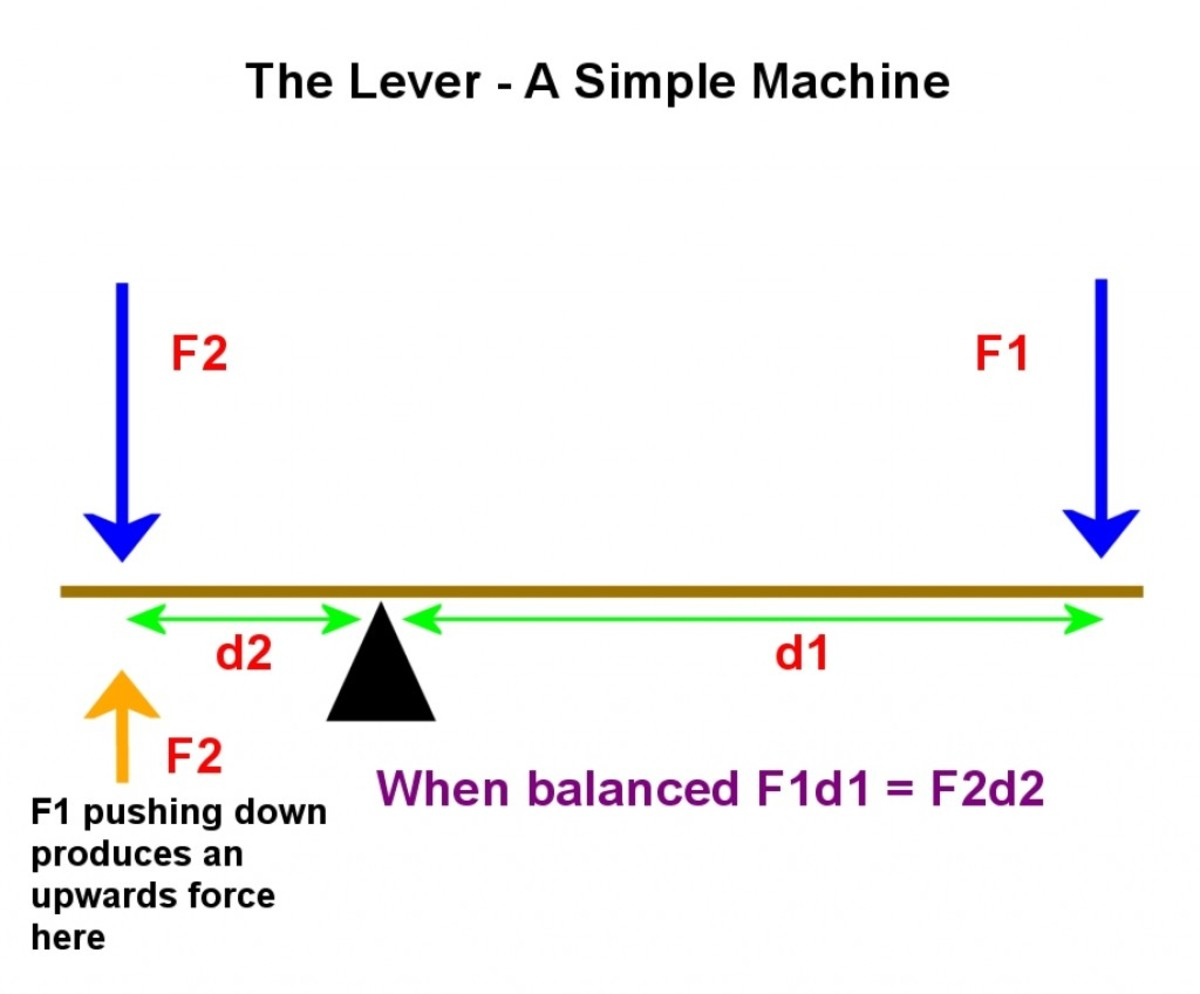When the lever is balanced, the force F1 produces an equivalent force of magnitude F2 (shown in orange). This balances F2 (shown in blue) acting downwards© Eugene Brennan

## The Law of the Lever

We can summarise the above reasoning into a simple equation known as the law of the lever:

Mechanical advantage = F2/F1 = d1/d2

## What is a Counterbalance Used For?

A counterbalance is a weight added to one end of a lever or other pivoting structure so that it becomes balanced (the turning moments clockwise and anti-clockwise are equalised). The weight of the counterbalance and its position relative to the pivot are set so that the lever can stay at any angle without turning. The advantage of a counterbalance is that a lever only has to be displaced and doesn't have to be physically lifted. So for instance a heavy road barrier could be raised by a human if it moves freely on its pivot. If there was no counterbalance, they would have to push down a lot harder on the barrier to lift the other end. Counterbalances are also used on tower cranes to balance the boom so that the crane doesn't topple over. Swing bridges use counterbalances to balance the weight of the swing section.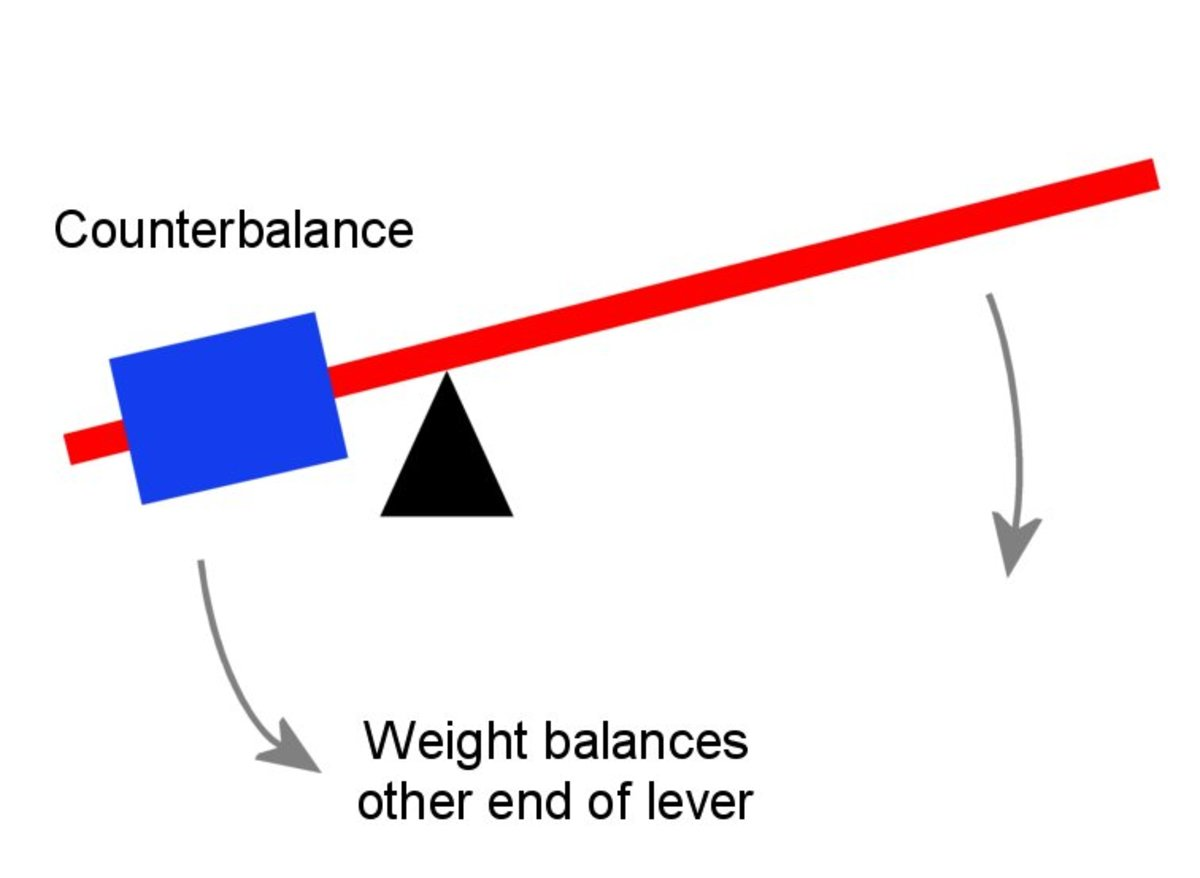A counterbalance used to balance a lever. These are often seen on road barriers where one end of the lever is much shorter than the other end.© Eugene Brennan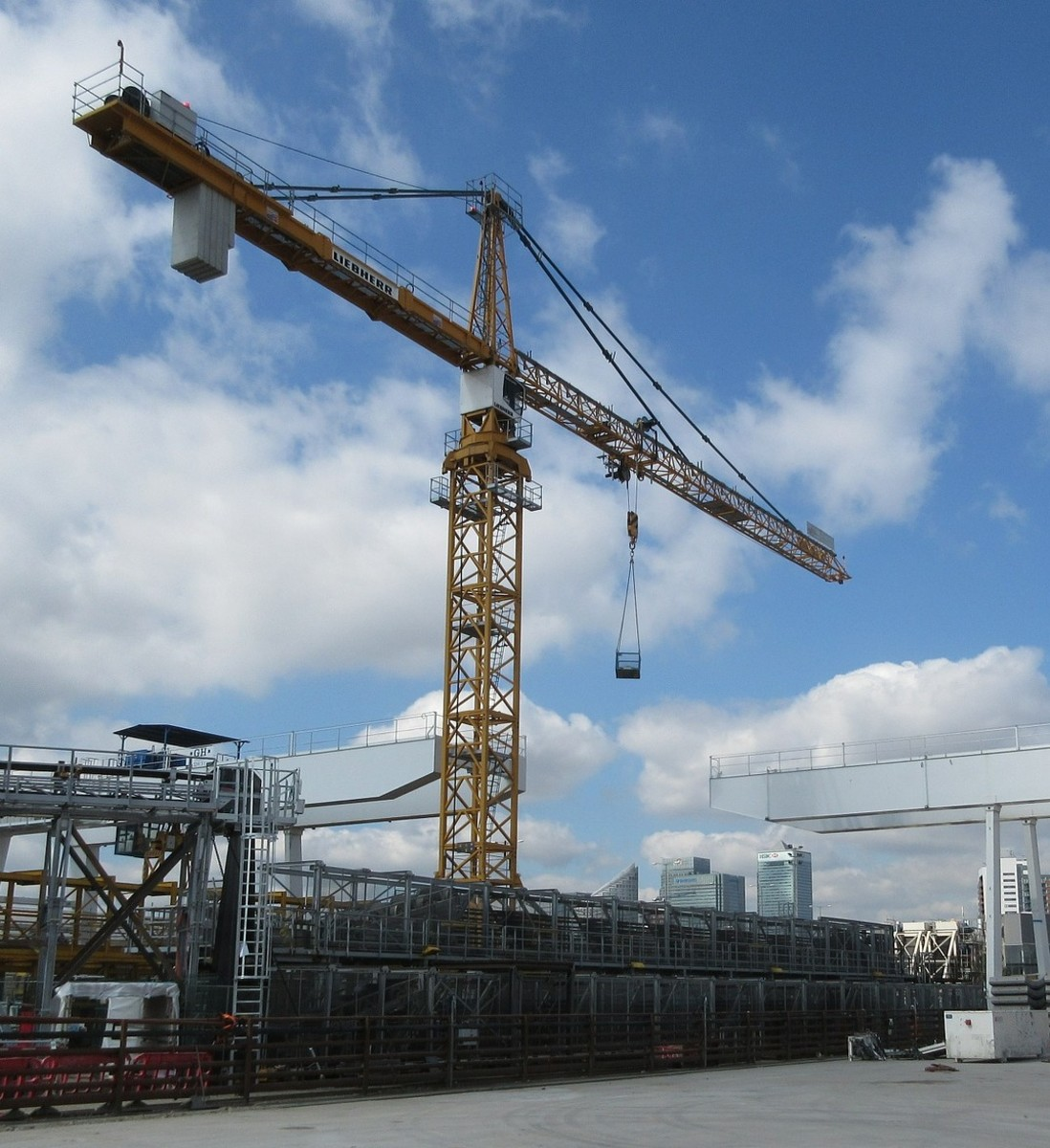A tower crane. The counterbalance consists of a collection of concrete slabs mounted near the end of the boom.Conquip, public domain image via Pixabay.com

## References

Hannah, J. and Hillerr, M. J., (1971) Applied Mechanics (First metric ed. 1971) Pitman Books Ltd., London, England.

Question: What are 3 examples of a lever?

Answer: Examples of a lever are a crowbar, nutcracker and broom.

Question: What is a lever and how is a lever useful?

Answer: A lever is one of the six simple machines. Levers can be used as links to connect the various moving parts of a machine, so, for instance, one part of a machine can move another part by pulling a link that can pivot at an intermediate point. Levers also take form in a variety of hand tools such as scissors, pliers, claw hammers and wheelbarrows. One of the main features of a lever that makes it useful is that it can have a mechanical advantage. This means that when a force is applied to one point on the lever (e.g. the end), another part of the lever can exert a larger force. So, for instance, a tool called a bolt cutter has long handles which give it a lot of mechanical advantage. This allows it to cut bolts. Another tool called a lopping shears also has long handles. This enables it to cut thick branches.

Question: But from an atomic level how can a small force at one end of the lever cause a bigger force at the other end (depending on the position of the pivot/fulcrum)?

Answer: There are some interesting discussions here:

https://physics.stackexchange.com/questions/22944/...